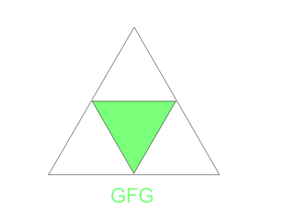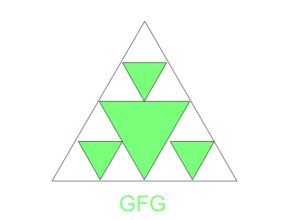# Count the total number of triangles after Nth operation

Given an equilateral triangle, the task is to compute the total number of triangles after performing the following operation N times.
For every operation, the uncolored triangles are taken and divided into 4 equal equilateral triangles. Every inverted triangle formed is colored. Refer to the below figure for more details.

For N=1 the triangle formed is:For N=2 the triangle formed is:Examples:

Input :N = 10
Output : 118097

Input : N = 2
Output : 17

## Recommended: Please try your approach on {IDE} first, before moving on to the solution.

Approach:

• At every operation, 3 uncolored triangles, 1 colored triangle and the triangle itself is formed
• On writing the above statement mathematically; count of triangles at Nth move = 3 * count of triangles at (N-1)th move + 2
• Therefore, initializing a variable curr = 1 and tri_count = 0
• Next, a loop is iterated from 1 to N
• For every iteration, the operation mentioned above is performed.
• Finally, the tri_count is returned
• Below is the implementation of the above approach:

## C++

 `#include ` `using` `namespace` `std; ` `// function to return the ` `// total no.of Triangles ` `int` `CountTriangles(``int` `n) ` `{ ` `    ``int` `curr = 1; ` `    ``int` `Tri_count = 0; ` `    ``for` `(``int` `i = 1; i <= n; i++) { ` `        ``// For every subtriangle formed ` `        ``// there are possibilities of ` `        ``// generating (curr*3)+2 ` ` `  `        ``Tri_count = (curr * 3) + 2; ` `        ``// Changing the curr value to Tri_count ` `        ``curr = Tri_count; ` `    ``} ` `    ``return` `Tri_count; ` `} ` ` `  `// driver code ` `int` `main() ` `{ ` `    ``int` `n = 10; ` `    ``cout << CountTriangles(n); ` `    ``return` `0; ` `} `

## Java

 `class` `Gfg { ` `    ``// Method to return the ` `    ``// total no.of Triangles ` `    ``public` `static` `int` `CountTriangles(``int` `n) ` `    ``{ ` `        ``int` `curr = ``1``; ` `        ``int` `Tri_count = ``0``; ` `        ``for` `(``int` `i = ``1``; i <= n; i++) { ` `            ``// For every subtriangle formed ` `            ``// there are possibilities of ` `            ``// generating (curr*3)+2 ` ` `  `            ``Tri_count = (curr * ``3``) + ``2``; ` `            ``// Changing the curr value to Tri_count ` `            ``curr = Tri_count; ` `        ``} ` `        ``return` `Tri_count; ` `    ``} ` ` `  `    ``// driver code ` `    ``public` `static` `void` `main(String[] args) ` `    ``{ ` `        ``int` `n = ``10``; ` `        ``System.out.println(CountTriangles(n)); ` `    ``} ` `} `

## Python

 `# Function to return the  ` `# total no.of Triangles ` `def` `countTriangles(n): ` `     `  `    ``curr ``=` `1` `    ``Tri_count ``=` `0` `    ``for` `i ``in` `range``(``1``, n ``+` `1``): ` `             `  `        ``# For every subtriangle formed ` `        ``# there are possibilities of  ` `        ``# generating (curr * 3)+2 ` `        ``Tri_count ``=` `(curr ``*` `3``) ``+` `2` `        ``# Changing the curr value to Tri_count ` `        ``curr ``=` `Tri_count ` `    ``return` `Tri_count ` `     `  `n ``=` `10` `print``(countTriangles(n)) `

## C#

 `using` `System; ` ` `  `class` `Gfg  ` `{ ` `    ``// Method to return the ` `    ``// total no.of Triangles ` `    ``public` `static` `int` `CountTriangles(``int` `n) ` `    ``{ ` `        ``int` `curr = 1; ` `        ``int` `Tri_count = 0; ` `        ``for` `(``int` `i = 1; i <= n; i++)  ` `        ``{ ` `            ``// For every subtriangle formed ` `            ``// there are possibilities of ` `            ``// generating (curr*3)+2 ` `            ``Tri_count = (curr * 3) + 2; ` `             `  `            ``// Changing the curr value to Tri_count ` `            ``curr = Tri_count; ` `        ``} ` `        ``return` `Tri_count; ` `    ``} ` ` `  `    ``// Driver code ` `    ``public` `static` `void` `Main(String[] args) ` `    ``{ ` `        ``int` `n = 10; ` `        ``Console.WriteLine(CountTriangles(n)); ` `    ``} ` `} ` ` `  `// This code is contributed by 29AjayKumar `

Output:

```118097
```

My Personal Notes arrow_drop_upCheck out this Author's contributed articles.

If you like GeeksforGeeks and would like to contribute, you can also write an article using contribute.geeksforgeeks.org or mail your article to contribute@geeksforgeeks.org. See your article appearing on the GeeksforGeeks main page and help other Geeks.

Please Improve this article if you find anything incorrect by clicking on the "Improve Article" button below.

Improved By : 29AjayKumar